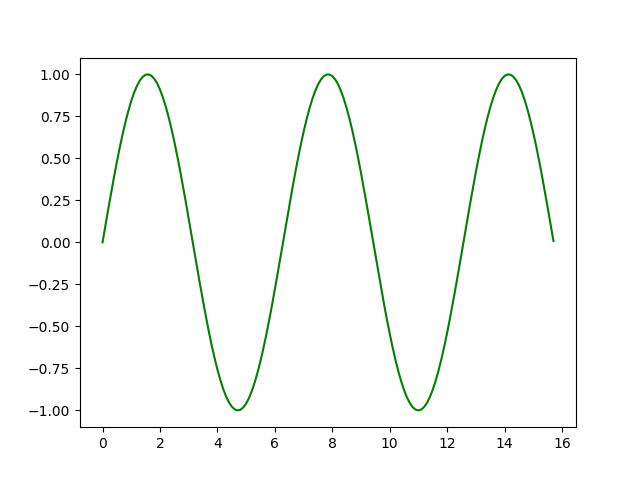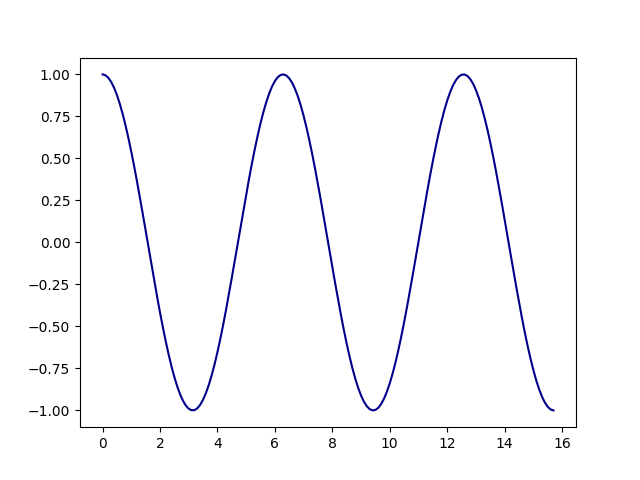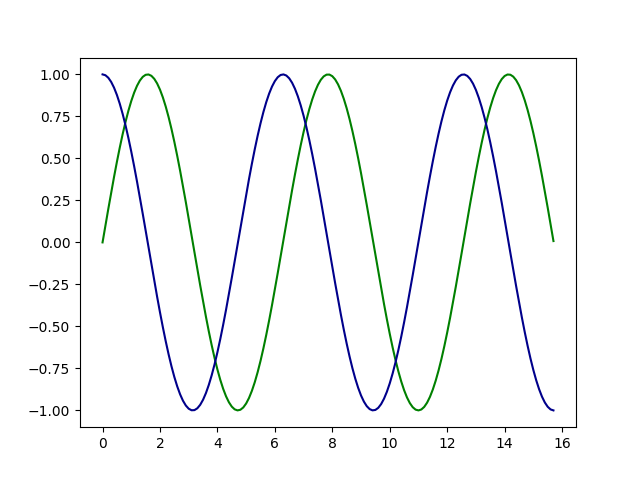Open in App
Not now

# Plotting Sine and Cosine Graph using Matplotlib in Python

• Last Updated : 22 Dec, 2022

Data visualization and Plotting is an essential skill that allows us to spot trends in data and outliers. With the help of plots, we can easily discover and present useful information about the data.In this article, we are going to plot a sine and cosine graph using Matplotlib in Python. Matplotlib is a Python library for data visualization and plotting, if you don’t have Matplotlib installed on your system, please install it before Plotting Sine and Cosine Graph using Matplotlib. One can install Matplotlib using the below command on the terminal or Jupyter notebook.

`pip install matplotlib`

### Plotting Sine Graph using Matplotlib in Python

Now let’s plot the sine curve using the sine function that is inbuilt into the NumPy library and plot it using Matplotlib.

Step 1: Import the necessary library for plotting.

## Python3

 `#Importing required library``import` `numpy as np``import` `matplotlib.pyplot as plt`

Step 2: Create a list or load your own data for Plotting Sine Graph.

## Python3

 `# Creating x axis with range and y axis with Sine``# Function for Plotting Sine Graph``x ``=` `np.arange(``0``, ``5``*``np.pi, ``0.1``)``y ``=` `np.sin(x)`

Step 3: Plotting Sine Graph with the created list or load data.

## Python3

 `# Plotting Sine Graph``plt.plot(x, y, color``=``'green'``)``plt.show()`

Output:Sine Curve using Matplotlib

### Plotting Cosine Graph using Matplotlib in Python

Now let’s plot the cosine curve using the cosine function that is inbuilt into the NumPy library and plot it using Matplotlib.

## Python3

 `# Importing required library``import` `numpy as np``import` `matplotlib.pyplot as plt` `# Creating x axis with range and y axis with Sine``# Function for Plotting Cosine Graph``x ``=` `np.arange(``0``, ``5``*``np.pi, ``0.1``)``y ``=` `np.cos(x)` `# Plotting coine Graph``plt.plot(x, y, color``=``'green'``)``plt.show()`

Output:Cosine Curve using Matplotlib

### Plotting Both Combined Sine and Cosine Graph

From the above two curves, one must think that both of them seem pretty similar. Let’s look at both of these curves on the same graph then we will be able to detect the difference between the graph of the two curves.

## Python3

 `# Importing required library``import` `numpy as np``import` `matplotlib.pyplot as plt` `# Creating x axis with range and y axis``# Function for Plotting Sine and Cosine Graph``x ``=` `np.arange(``0``, ``5``*``np.pi, ``0.1``)``y1 ``=` `np.sin(x)``y2 ``=` `np.cos(x)` `# Plotting Sine Graph``plt.plot(x, y1, color``=``'green'``)``plt.plot(x, y2, color``=``'darkblue'``)``plt.show()`

Output:Sine and Cosine Curve using Matplotlib

My Personal Notes arrow_drop_up